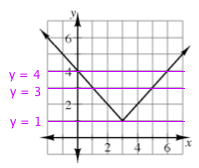### Home > AC > Chapter 12 > Lesson 12.1.3 > Problem12-27

12-27.

Use the graph of $f ( x ) = | x - 3 | + 1$ in problem 12-26 to solve the equations and inequalities below. It may be helpful to copy the graph onto graph paper first.

1. $| x - 3 | + 1 = 1$

1. $| x - 3 | + 1 \leq 4$

1. $| x - 3 | + 1 = 3$

1. $| x - 3 | + 1 > 2$

Remember that with absolute values, there may be $2$ possible solutions.

Draw line $y=1$ onto the graph and find the intersection point(s).

$x = 3$

See part (a).